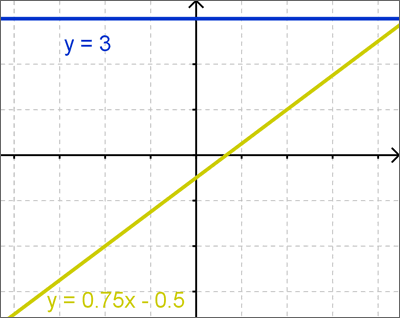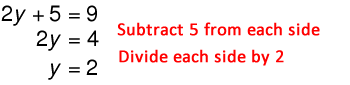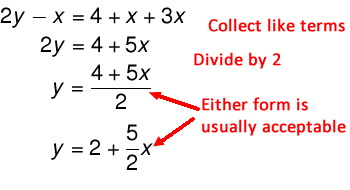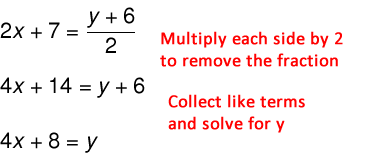# What makes an equation linear?

### Simple Definition of Linear Equation:

An equation that forms a straight line on a graph.

More precisely, a linear equation is one that is dependent only on constants and a variable raised to the first power. For example, $$y=6x+2$$ is linear because it has no squares, cubes, square roots, sines, etc. Linear equations can always be manipulated to take this form:

$$ax+b=0$$

You won't always see linear equations written exactly like that, but keep in mind that we can manipulate equations to put them in a particular form if necessary.

Linear equations are often written with more than one variable, typically x and y. Such equations will have many possible combinations of x and y that work. When those points (known as coordinate pairs) are plotted on an x-y axis, they will form a straight line. Let's take a look at this graphically below. The two equations drawn are linear. Note that one is in the form $$y=3$$ (it is dependent on just a constant, 3), and the other equation is $$y=0.75x - 0.5$$ (a linear term and a constant).### How do I know if an equation is linear?

Does the equation (or function) include any squared terms? How about other terms with exponents other than 1 (or, technically, zero)? If the function has no terms with an order higher than 1 (a fancy way of saying exponent) then it is linear!

### What if it has a log or trig function, etc.?

These are not linear terms. Simply, they aren't constants (regular numbers) or variables with an exponent of 1, so the function isn't linear. If we could write sin(x) or log(x) as something linear like $$2x+3$$ then we'd do that instead of using complicated non-linear functions like sine and log! Of course, if you haven't covered these concepts in your class yet, don't even worry about it.

### So, how do I solve a linear equation?

$$y=4$$

It's a linear equation, and it's already solved for y! That's easy... there's nothing to do. But this rather trivial example does show us that linear equations can be quite simple, and also shows us our goal: rewrite the equation so that the variable we are solving for is on one side, and everything else is on the other side.

Taking it a tiny step forward:

$$y+2=4$$

With this equation we simply have to subtract 2 from both sides in order to put our equation in a solved form, with y=2. Solving any linear equation is just a matter of performing operations on both sides of the equals sign until the equation is in the form you want (usually solved for a single variable, like X or Y). The steps are shown in detail below:

$$y+2=4$$ $$y+2-2=4-2$$ $$y+0=2$$ $$y=2$$

### What about more complicated equations?

Fortunately with linear equations, the steps are always relatively straightforward. There's no one way to do it, and with time you'll be able to think through a linear equation without really having to write down every step. Try the following approach to solve the equations and see if it works for you:

1. Collect like terms -- this means to gather all the x's together, all the y's together, and all the regular numbers (known as constants) and add them up separately. For example, the expression $$4x+2y+3x-5+10$$ becomes $$7x+2y+5$$. Remember that you can add, subtract, multiply, or divide so long as you do it to both sides of an equation.
2. Isolate the variable you wish to solve for -- If the problem asks you to solve for y, you need to get y on one side of the equals sign and all the other stuff on the other side. This is where you might go from $$2y - 6 = 4$$ to $$2y = 10$$.
3. Remove any coefficient remaining on that variable -- if your answer after step 2 looks like $$5y = 7x - 10$$, just divide both sides by 5 to get $$y=\frac{7x}{5} - \frac{10}{2}$$.

### Let's look at some examples of solving linear equations.

One thing to keep in mind is that you can't always solve the equation to something definite like y=5. It's perfectly ok to have y=x+5, and it just means that y depends on x. In fact, there's exactly one value of y for each value of x, all of which make points that lie on a straight line (like I showed back at the beginning).

### Example 1:

Solve for y: $$2y+5=9$$If you substitute 2 for y back in the original problem, you get 9=9, so it's right!

### Example 2:

Solve for y: $$2y-x=4+x+3x$$### Example 3:

Solve for y: $$2x+7=\frac{y+6}{2}$$### Wrapping it all up

Remember that linear equations are inherently simple -- don't try to overthink things! They consist only of linear terms (like 3x, 2y, y/2, etc.) and constants. If you get stuck trying to simplify or solve a problem, just remember to take it one step at a time. Collect like terms by combining all of your variables separately, then isolate the variable you wish to solve for, and finally perform any additional math required so you are left with just "y=" or "x=" on one side of the equation.